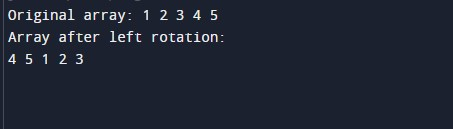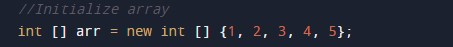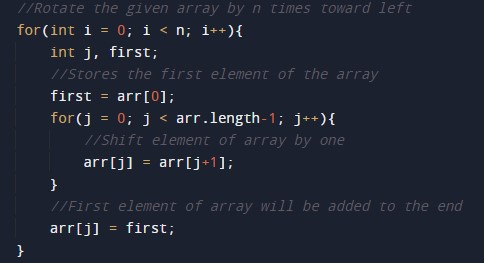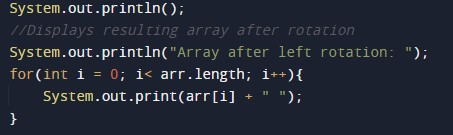# Java Program to left rotate the elements of an array

In this tutorial you will learn about the Java Program to left rotate the elements of an array and its application with practical example.

In this tutorial, we will learn to create a Java Program to left rotate the elements of an array using java programming.

## Prerequisites

Before starting with this tutorial, we assume that you are the best aware of the following Java programming concepts:

• Java Operators.
• Basic Input and Output function in Java.
• Class and Object in Java.
• Basic Java programming.
• If-else statements in Java.
• For loop in Java.

## Rotate an array left, means?

The array is said to be left rotated if elements in an array are moved to its left >> by one(1) position.

Example:

Array⇾ [1, 2, 3, 4, 5].
Rotate left ⇾ [5,1,2,3,4].

## Java Program to left rotate the elements of an array

In this program, we will shift the left element in an array using for loop. We would first declare and initialized the required variables. Next, we will shift elements of an array. Let’s have a look at the code.

## Output

Left  rotated array[]In the above program, we have first declared and initialized a set of variables required in the program.

• arr[]= it will hold array values
• first =  it will hold rotation position.
• i       = for iteration of the program.
• n = it will hold the integer value for left side.

After declaring variables we initiate values in  an array[]After that, we will call left Rotate(n , first ) where we pass two arguments one array and another is rotated by value i.e. how many times rotate the array to its Left.In an array, if Lotby is “1” then, elements of an array are moved to its Left by one position it means the first element of the array takes the second(2) position, the second element will be moved to the third position, and so on. As shown down below⇾

Array⇾ [1, 2, 3, 4, 5].
Rotate Left ⇾ [4, 5, 1, 2, 3].

Finally, print the Left rotated array.In this tutorial we have learn about the Java Program to left rotate the elements of an array and its application with practical example. I hope you will like this tutorial.